Skip to content ↓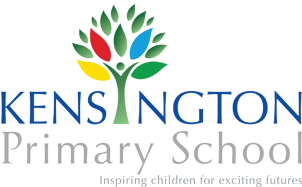# Kensington Primary School

••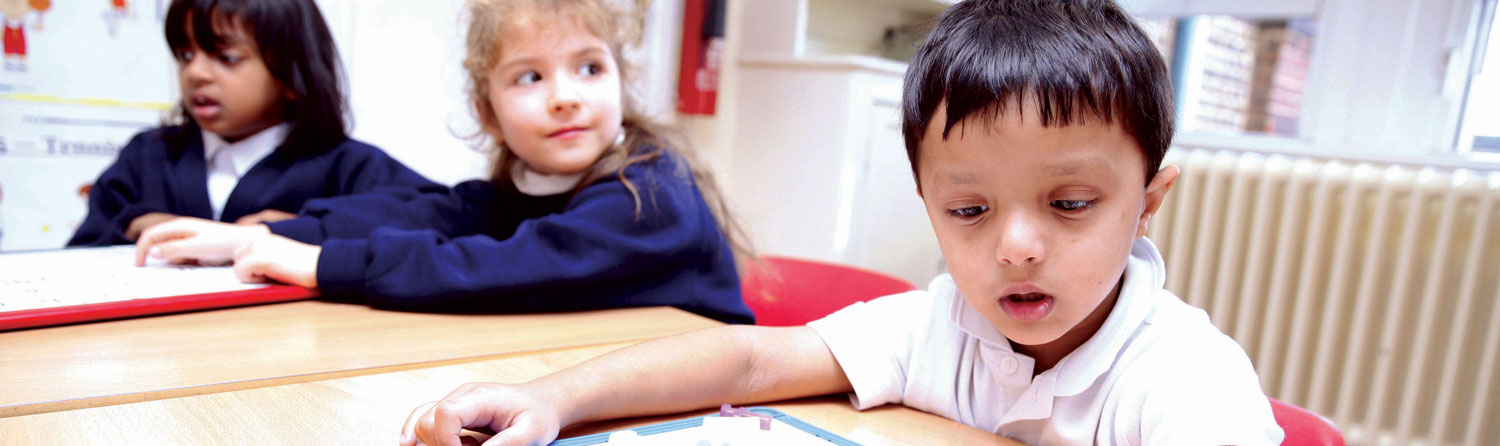•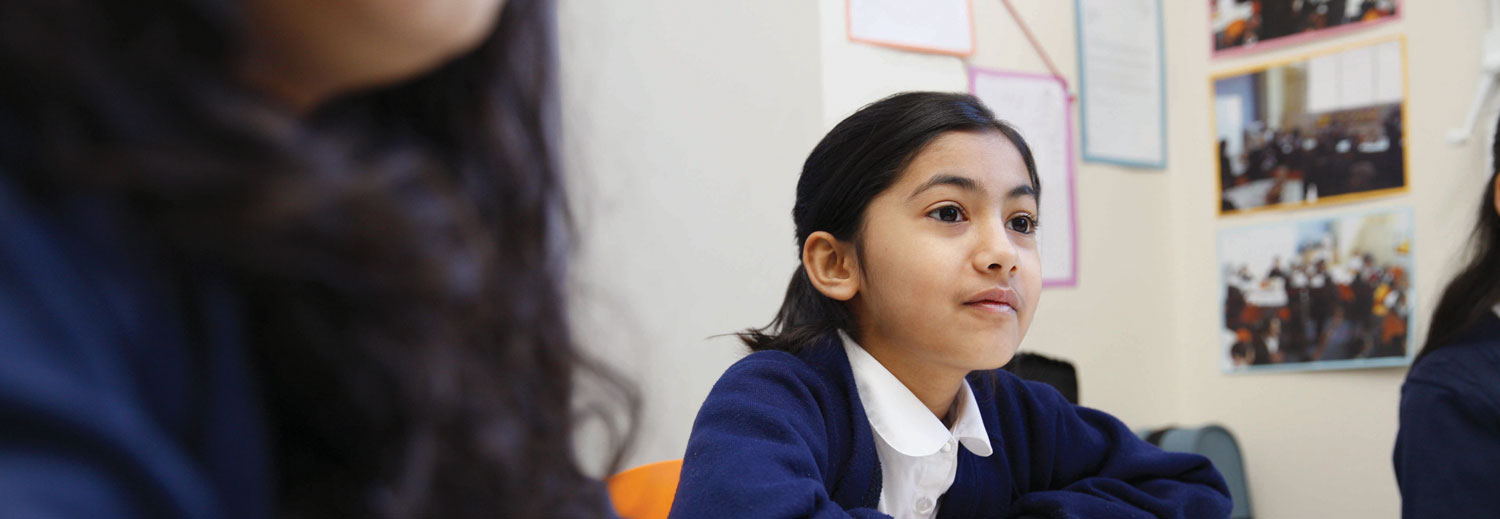•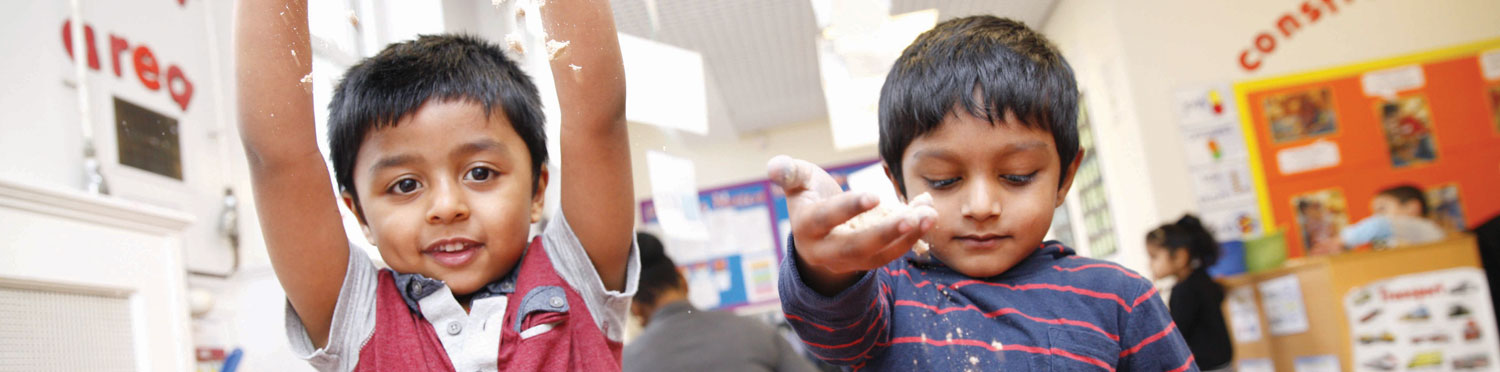•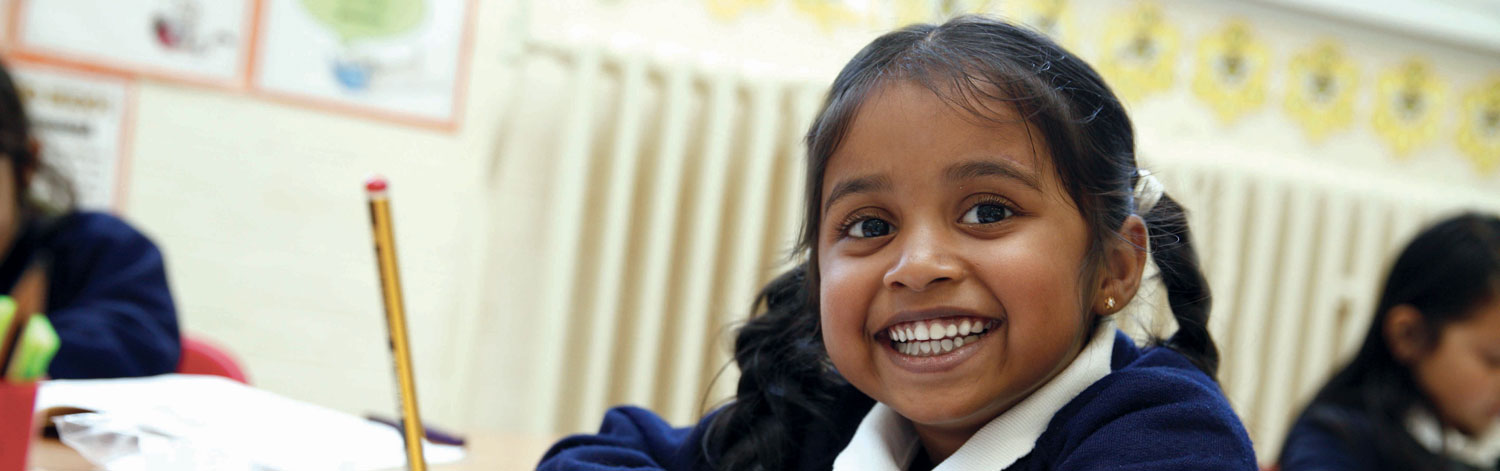# Maths

The teaching of maths has evolved over the last few years. In particular, the focus has been on looking at the success in countries such as Singapore and trying to understand what lessons we can learn. At Kensington, we follow some key principles in mathematics that are aimed at developing confident, fluent mathematicians.

### Objects and pictures

Children use concrete manipulatives (objects) and pictorial representations (pictures), before moving to abstract symbols (numbers and signs). This is called: CPA (Concrete-Pictorial-Abstract).

When we use number in any situation, we have a mental 'picture' of what this looks like. If you are very confident with number, this picture is so embedded that you hardly see it anymore: but it is there. By using concrete manipulatives we are giving children a physical representation that, over time and with practise, will become their mental picture.

144 Maths Resources can be found below

### Language development

The way that children speak and write about mathematics has been shown to have an impact on their success. We use a carefully sequenced, structured approach to introduce and reinforce mathematical vocabulary. Every lesson includes opportunities for children to explain or justify their mathematical reasoning.

### Problem solving

Mathematical problem solving is at the heart of our approach – it is both how children learn maths, and the reason why they learn maths. By accumulating knowledge of mathematical concepts, children can develop and test their problem solving in every lesson.

We run regular workshops for parents. If you would like to find out more, please sign-up for our next workshop..

### 20/20 and 144 Clubs

Having a secure knowledge of the key number facts - number bonds and timestables - is at the heart of developing mathematical confidence. To support children with this Kensington has the 20/20 and 144 clubs.

20/20: For children in KS1. To become a member they will be required to have secure knowledge of their number bonds and basic facts up to 20.

144 Club: For children in KS2. To become a member they will be required to have secure knowledge of their basic facts and times tables up to 12 x 12.

Students will be tested once every half term and if they pass they will become a member of either the 20/20 Club or the 144 Club. Their name will be displayed on a display board in the hall.

## The Year 1 Learner

By the end of year 1, children begin to solve simple problems involving addition and subtraction in familiar contexts such as going shopping, using a range of concrete materials, symbols, images and pictures. They begin to use what they know to tackle problems that are more complex and provide simple reasons for their opinions.

Number Counting and understanding numbers
Children will identify and represent numbers using objects, pictures and models, such as the number line, and use ‘equal to, more than, less than (fewer), most and least.’ Children will accurately count numbers to, and across, 100 forwards and backwards from any given number with increasing understanding. They count, read, write and order numbers in numerals up to 100 and from 1 to 20 in words. When given a number, they can identify one more and one less. They can count in multiples of twos, fives and tens.

Calculating
Children will understand known addition and subtraction facts within 20, including zero. They will demonstrate an understanding of multiplication and division through grouping and sharing using concrete, pictorial representations and arrays (2, 5 and 10). They understand doubling and halving small quantities.

Fractions
Through exploration, talks tasks and the use of concrete materials children will find and name half and one quarter of objects, shapes and quantities.

Measurement
Children will begin to measure using non-standard units (finger widths, blocks etc.) moving to standard units of measure (e.g. cm) using tools such as a ruler, weighing scales and containers. They will begin to record and compare measurements such as lengths and heights, mass and weight, capacity and volume using language such as long / short; heavy / light; full / half-full / empty. They will tell the time to the hour, half past the hour and be able to sequence events in chronological order using precise language (for example, before and after, next, first, today etc.). Children will recognise and know the value of different denominations of coins and notes.

Geometry
Children will recognise and name common 2-D shapes, e.g. rectangles (including squares), circles and triangles, and 3-D shapes, e.g. cuboids (including cubes, pyramids and spheres) in different orientations and sizes. They will describe position, direction and movement, including whole, half and three quarter turns.

## The Year 2 Learner

By the end of year 2, children will solve problems with one or a small number of simple steps. Children will discuss their understanding and begin to explain their thinking using appropriate mathematical vocabulary, hands-on resources and different ways of recording. They will ask simple questions relevant to the problem and begin to suggest ways of solving them.

Number Counting and understanding numbers
Children will develop their understanding of place value of numbers to at least 100 and apply this when ordering, comparing, estimating and rounding. Children begin to understand zero as a place holder as this is the foundation for manipulating larger numbers in subsequent years. Children will count fluently forwards and backwards up to and beyond 100 in multiples of 2, 3, 5 and 10 from any number. They will use concrete materials and pictorials to help them understand and apply their knowledge of place value in two digit numbers, representing the numbers in a variety of different ways.

Calculating
Children learn that addition and multiplication number sentences can be re-ordered and the answer remains the same (commutativity) such as 9+5+1= 5+1+9. They learn that this is not the case with subtraction and division. They solve a variety of problems using mental and written calculations for +, -, x, ÷ in practical contexts. These methods will include partitioning which is where the number is broken up into more manageable parts (e.g. 64 = 60 + 4 or 50 + 14), re-ordering (e.g. moving the larger number to the beginning of the number sentence when adding several small numbers) and using a number line. Children will know the 2, 5 and 10 times tables, as well as the matching division facts (4 x 5 = 20, 20 ÷ 5 = 4) and can recall them quickly and accurately. They apply their knowledge of addition and subtraction facts to 20 and can use these to work out facts up to 100.

Fractions including decimals
Throughout year 2, children will develop their understanding of fractions and the link to division. They explore this concept using concrete materials, pictures, images and concrete materials. They will solve problems involving fractions (e.g. find 1/3 of the hexagon or 1⁄4 of the marbles) and record what they have done. They will count regularly and fluently in fractions such as 1⁄2 and 1⁄4 forwards and backwards and, through positioning them on a number line, understand that some have the same value (equivalent) e.g. 1⁄2 = 2/4.

Measurement
Children will estimate, choose, use and compare a variety of measurements for length, mass, temperature, capacity, time and money. By the end of year 2, they will use measuring apparatus such as rulers accurately. They will use their knowledge of measurement to solve problems (e.g. how many ways to make 50p). They extend their understanding of time to tell and write it on an analogue clock to 5 minute intervals, including quarter past / to the hour. They will know key time related facts (minutes in an hour, hours in a day) and relate this to their everyday life.
Geometry
Children will identify, describe, compare and sort common 2-D and 3-D shapes according to their properties (sides, vertices, edges, faces) and apply this knowledge to solve simple problems. They develop their understanding by finding examples of 3-D shapes in the real world and exploring the 2-D shapes that can be found on them (e.g. a circle is one of the faces on a cylinder). Children begin to describe position, direction and movement in a range of different situations, including understanding rotation (turning through right angles clockwise and anti-clockwise). They use their knowledge of shape in patterns and sequences.

Statistics
Children sort and compare information, communicating findings by asking and answering questions. They will draw simple pictograms, tally charts and tables.

## The Year 3 Learner

By the end of year 3, children will talk about their mathematics in full sentences using key vocabulary. They will use the numbers they are familiar with, applying their understanding of number, measures and shape to a greater range of problems. They will make decisions about calculations and information that is needed to solve problems, for example when a recipe for two people needs to be doubled to make a recipe for four. Children will be expected to prove their thinking through the use of concrete materials, pictures, jottings and conversations. They will be encouraged to pose their own questions, working in an organised way to solve them, which will help pupils to identify common patterns or any errors more easily.

Number
Counting and understanding numbers
Children will be very familiar with numbers that have 3 digits and will have experienced many opportunities to order, compare and show them in different ways using apparatus such as a tape measure, a 100 grid or money. Using their understanding of place value (how the value of each digit changes depending on its position in the number), children will be able to partition (break and make) numbers in different ways e.g. 234 = 200 and 30 and 4; 100 and 100 and 20 and 10 and 4; or 200 and 20 and 14. They will develop a secure understanding of numbers up to 1000 and will count beyond it in 1s, 10s and 100s. They will use this counting to help find 10 or 100 more than any given number. Children will be introduced to numbers with one decimal place and will count up and down in tenths; share groups of objects or shapes into tenths and represent these in pictures and using hands-on resources. Children will count forwards and backwards from 0 in steps of 4, 8, 50 and 100 and link this to multiplication and division. They will also count in 3s to help maintain their fluency from Year 2.

Calculating
Children will continue to develop their mental calculation skills to add and subtract combinations of three-digit numbers e.g. 248 +/- 8; 319 +/- 40; 428 +/- 200. They will develop their range of strategies using jottings (sketches and notes to help them remember the steps) and number lines to help them understand how each calculation works. Children will share their methods with others to help them see which work best, are quickest and most accurate. Children will understand the importance of estimation when calculating to see if their answer is reasonable or not. They will recall their multiplication and division facts for 3, 4 and 8x tables and be supported to see the links between the 2, 4 and 8x tables. They explore patterns and rules for the times tables they learn and will use pictures and objects to support their understanding. They will also learn that multiplication can be done in any order e.g. 3 x 4 x 2 = 2 x 3 x 4.

Children will be introduced to more formal methods of recording addition and subtraction, including column methods. They will use concrete materials and pictorials to secure their understanding of these methods. This will be applied to numbers up to three digits. Children who become very adept at these calculations will be stretched through problems such as those involving missing numbers or digits so that they know when, if and why they need to use these methods. Children will develop their understanding of multiplication and division and apply their times table knowledge to multiply 2-digit by 1-digit numbers using the skills of partitioning (breaking and making numbers). For example, 43 x 5 can also be thought of as 40 x 5 and 3 x 5 or (4 x 5 x 10) + (3 x 5). They will move from informal methods of calculating multiplication and division to formal written methods i.e. short column multiplication and be supported by using concrete materials and pictorials.

Fractions
Children will develop their understanding of fractions and decimals and will be introduced to tenths. They will count and understand tenths as ten equal parts as well as through dividing sets of objects into ten equal parts / groups. They will find and write fractions of objects using their multiplication tables knowledge, e.g. 1/5 of a group of 20 buttons can be solved by 20 ÷ 5 = 4, and will continue to explore equivalent fractions using diagrams to explain their understanding e.g. 2/4 is equivalent to or of equal value to 4/8. They will also begin to add and subtract fractions where the denominator is the same e.g. 4/6 + 1/6 = 5/6.

Measurement
Children will continue to measure, compare, add and subtract measurements and progress to mixed units e.g. expressing amounts as litres and millilitres – 2 litres 400ml. They will measure the perimeter of 2-D shapes and will continue to add and subtract amounts of money including giving change. Children will estimate and read time to the nearest minute on analogue and digital clock faces. They will be introduced to the Roman numerals I to XII to help with this. Problem solving and calculating with time will involve working within contexts such as comparing the duration of events such as the length of favourite television programme or journeys to school. They will use language with increasing accuracy, such as seconds, minutes and hours; o’clock, a.m. / p.m., morning, afternoon, noon and midnight. They will need to recall the number of seconds in a minute and the number of days in each month, year and leap year.

Geometry
Children will accurately draw 2-D shapes with rulers measuring sides accurately. They will make 3-D shapes to help them understand how they are composed and will recognise 3-D shapes in a range of places and contexts (e.g. buildings, packages) and use correct mathematical vocabulary to describe them. They will learn what a right angle is and know that two right angles make a half-turn, three make three quarters of a turn and four a complete turn as well as identify whether angles are greater than or less than a right angle. They will also be able to identify horizontal and vertical lines and pairs of perpendicular (␣) and parallel lines (=).

Statistics
Children will collect, organise, answer and pose questions about information using bar charts, pictograms and tables to answer questions such as ‘how many more children prefer football to cricket?’.

## The Year 4 Learner

By the end of year 4, children will apply their understanding of mathematics to solve a wide variety of problems with more than one step and be expected to prove their thinking through the use of concrete materials, pictures, jottings and conversations using key vocabulary. They will continue to make connections between different areas of mathematics and ask their own questions, working in an organised way to find solutions, which help them identify common patterns or any errors more easily.

Number Counting and understanding numbers
Children will be very familiar with numbers that have up to 4 digits and will be able to order and compare by showing them in different ways such as on a tape measure or using concrete materials. Using their understanding of place value (how the value of each digit changes depending on its position in the number), children will be able to partition (break and make) numbers in different ways e.g. 2345 = 2000 and 300 and 40 and 5 but could also represent this as 1000 and 1000 and 200 and 100 and 40 and 5 or 2000 and 200 and 145. They will work with numbers securely up to 10,000 and may begin to count beyond in 1s, 10s, 100s and 1000s. They will use this to help them find 10, 100 or 1000 more or less than any given number. They will multiply and divide whole numbers by 10 and 100 and understand that this changes the value of each digit rather than ‘just adding a 0’. They will develop their understanding to decimal hundredths, comparing and ordering these using contexts such as money. Children will also learn about the pattern to find any Roman numeral to 100.

Children will develop their expertise when counting forwards and backwards from 0 to include multiples of 6, 7, 9 and 25; decimals with up to 2 places and fractions. They will be able to fluently count in tenths, hundredths and simple fractions. They will develop their understanding of negative numbers through counting backwards through 0. Children will be able to recognise and describe number patterns and relationships including multiples (e.g. 3, 6, 9, 12 are multiples of 3) and factor pairs (e.g. 1 and 12, 2 and 6, 3 and 4 are all factor pairs for 12) for known times tables.

Calculating
Children will develop various strategies for solving +, -, x, ÷ calculations mentally, using jottings when appropriate and for checking that their answers are sensible. Children will be encouraged to share their methods with others to help them see which work best, are quickest and most accurate. Over the course of the year, children will become fluent in all multiplication and division facts up to 12 x 12 and apply these facts to other problems e.g. 232 x 7 = (200 x 7) + (30 x 7) + (2 x 7). Children will use the = sign to demonstrate equal value e.g. 3 x 8 = 48 ÷ 2 and solve missing number problems e.g. 3 x ? = 48÷2. They will explore patterns and rules for the times tables they learn and use concrete materials and pictorials to support their understanding.

Children will be required to solve problems accurately using the column addition and subtraction methods for numbers with up to 4-digits and show and explain how the methods work. They will use a range of concrete materials to secure their understanding of these. This will include addition and subtraction calculations with different numbers of digits (such as 1286 + 357); and numbers containing 0s (such as 8009 – 3231). They will use formal written methods of short multiplication and short division for two and three digit numbers by a single digit. Children who become very adept at these types of calculations will be stretched through problems such as those containing missing numbers and digits so that they know when, if and why they need to use the methods.

Fractions including decimals
Children will develop their understanding of fractions by comparing to, or finding a part of, the whole. Through the use of concrete materials, pictorials and/or jottings, such as a number line, children will add and subtract two fractions with the same denominator (e.g. 2/3 + 2/3). Children will solve problems involving fractions such as ‘find 3⁄4 of 20 litres’ using their knowledge of multiplication and division and through practical equipment. Children will secure their understanding that fractions and decimals are different ways of expressing numbers and proportions.

Measurement
Children secure their understanding of place value and decimals to record measurements accurately. They use their understanding of multiplying and dividing by 10, 100 and 1000 to convert between different units of measure of length (km, m, cm, mm), weight (kg, g) and money (£ and p). Children will link their understanding of area to multiplication and describe how to find the perimeter of a rectangle quickly. Children will read and write the time accurately using analogue and digital clocks, including clocks with Roman numerals. They will convert between units of time (hours, minutes and seconds). Children estimate, compare, calculate and solve a variety of problems involving all units of measurement.

Geometry
Children will extend their knowledge of shape to include more unusual quadrilaterals (four-sided shapes) and triangles. They will use increasingly more specific vocabulary such as parallelogram, rhombus and trapezium; scalene and isosceles. They refine their understanding of symmetry and solve problems where the shape is not displayed in its usual way (e.g. it might be on its side). Children find and name different angles and use this information to decide if a shape is regular or irregular. Children describe position and movement on a grid as co-ordinates and will plot points to draw 2-D shapes.

Statistics
Children will complete, read and interpret information on bar charts; they will solve problems that involve finding information in charts, tables and graphs; including time graphs.

## The Year 5 Learner

By the end of year 5, children will apply their mathematical experiences to explore ideas and raise relevant questions, constructing complex explanations and reasoned arguments using concrete materials and pictorials to support their findings. They will be able to solve a wide variety of complex problems, which require sustained concentration and demand efficient written and mental methods of calculations. These will include problems relating to fractions, scaling (times as many), converting between units of measure and employ all four operations (+, -, x, ÷).

Number Counting and understanding numbers
Children will extend and apply their knowledge of place value for numbers up to one million, rounding, estimating and comparing them (including decimals and negative numbers) in a variety of situations. They are introduced to powers of ten and are able to count forwards or backwards from any number (for example, -50, -5... 5, 50, 500, 5000...). Through investigations, they will discover special numbers including factors, primes, square and cube numbers.

Calculating
Children will be fluent in a wide range of mental calculation strategies for all operations and will select the most appropriate method dependent on the calculation. They apply their knowledge of place value fluently to multiply and divide numbers (including decimals) by 10, 100 and 1000. When mental methods are not appropriate, they use formal written methods of addition and subtraction accurately. They continue to develop their understanding of the formal methods through the use of concrete materials, pictorials and known facts within long multiplication (up to 4 digit numbers by 2 digit numbers e.g. 2345 x 68) and short division (up to 4 digit numbers by 1 digit number e.g. 2345 ÷ 7) which may result in remainders. They solve multi- step problems in meaningful contexts and decide which operations to use.

Fractions including decimals and percentages
Children will secure their strong understanding that fractions express a proportion of amounts and quantities (such as measurements), shapes and other visual representations. Children will extend their knowledge and understanding of the connections between fractions and decimals to also include percentages. They will be able to derive simple equivalences (e.g. 67% = 67/100 = 0.67) and recall percentage and decimal equivalents for 1⁄2, 1⁄4, 1/5, 2/5, 4/5 and fractions with a denominator of a multiple of 10 or 25 (e.g. 25% = 25/100).

They will order, add and subtract fractions, including mixed numbers and those whose denominators are multiples of the same number,
for example        +       =       +       =       =       .

Using mathematical equipment, images and models, they multiply proper fractions and mixed numbers by whole numbers. Children will continue to develop their understanding of fractions as numbers, measures and operators by finding fractions of numbers and quantities in a variety of real life situations.

Measurement
Through a wide variety of practical experiences and concrete materials, children will extend their understanding of measurement. They will  convert larger to smaller related units of measure and vice-versa including length, capacity, weight, time and money. Children will convert between imperial (such as inches, pints, miles) and metric units (such as centimetres, litres, kilometres). Children will measure, calculate and solve problems involving perimeter of straight-sided, right-angled shapes (rectilinear) and learn to express this algebraically such as, 4 + 2b = 20. They find and measure the area of these shapes with increasing accuracy. They begin to estimate volume.

Geometry
Children will measure, identify and draw angles in degrees, developing a strong understanding of acute, obtuse, reflex and right angles. They use this knowledge to find missing angles and lengths in a variety of situations, including at a point, on a straight line and within a shape. Children will move (translate), reflect shapes and describe their new positions. Language will be used with increasing sophistication to compare and classify shapes based on their properties and size. They will be able to visualise 3-D shapes from 2-D diagrams. They will use their understanding or shapes to solve problems.

Statistics

Children will complete, read and solve comparison, sum and difference problems using information presented in graphs, charts and tables, including timetables. They begin to decide which representations of data are the most appropriate and are able to justify their reasons.

## The Year 6 Learner

By the end of year 6, children will structure their own investigations and solve a wide variety of increasingly complex problems. They will independently develop their own lines of enquiry and be expected to prove their solutions in a variety of ways including algebra, negative proof (use a counter example to prove the rule) and be able to communicate their results using accurate mathematical language, concrete materials and pictorials. Children will demonstrate secure knowledge and confidence to talk in depth about mathematical concepts and explain their solutions, decisions and reasoning.

Number Counting and understanding numbers
Children extend and apply their knowledge of place value for numbers up to and beyond one million (including decimals and negative numbers) in a variety of situations. Special numbers are extended to include common factors, common multiples and a deeper understanding of prime numbers. Children will be able to round numbers and identify what degree of accuracy is appropriate.

Calculating
Children will be fluent in a wide range of mental and formal written calculation strategies for all operations, extending to long division (four digit numbers by two digit numbers) by the end of the year. They will apply estimation in a range of ways. Through investigations, they explore the effect of the order of operations including the use of brackets.

Fractions including decimals and percentages
Children recall and using equivalences between simple fractions, decimals and percentages. Additionally, they are able to express fractions in their simplest form and calculate the decimal equivalent, for example 3/8 = 3 ÷ 8 = 0.375. Applying this understanding of equivalent fractions, children will order, add and subtract fractions (including mixed numbers and those with different denominators) by the end of the year e.g. 1/3 + 1/4 + c = 1. Using concrete materials, resources and images, they will multiply and divide proper fractions and mixed numbers by whole numbers. Children will solve problems involving the calculation of percentages linked to a variety of real life situations.

Ratio and proportion
Pupils explore ratio and proportion through real life experiences such as changing the quantities in recipes (scaling), scale drawings and maps.

Algebra
Throughout their primary experience children will have encountered algebra in a number of different situations, which are drawn together and formalised in year 6. By the end of the year, they will confidently use symbols and letters to represent variables and unknowns in mathematical situations that they already understand, for example, simple formula and equivalent expressions a+b = b+a. Children will describe number sequences and missing number calculations.

Measurement
Through investigation and problem solving, children will convert between a range of measurement units (including both imperial and metric). Calculation of perimeter and area is extended to include parallelograms and triangles. Additionally, they will explore the relationship between area and perimeter. They will know how to calculate, estimate and compare volume of cubes and cuboids identifying when it is appropriate to use formula.

Geometry
Children will draw 2-D and build 3-D shapes with accuracy using given dimensions and angles. They will create nets of common 3-D shapes. They will consolidate their knowledge of angles within shapes and extend it to find missing angles in triangles, quadrilaterals and regular polygons. Children name parts of circles, including radius, diameter and circumference, and explore the relationships between these elements. Children will use four quadrant co-ordinate grids to describe positions, draw and translate simple shapes. Using their knowledge of the properties of shape, they will be able to predict missing co-ordinates and express these algebraically.

Statistics
Children will increase their knowledge of different data representations to include interpreting and constructing pie charts (using their knowledge of angles, fractions and percentages) and line graphs (e.g. miles to km conversion). They will know when it is appropriate to use the mean as an average and how to calculate it.

•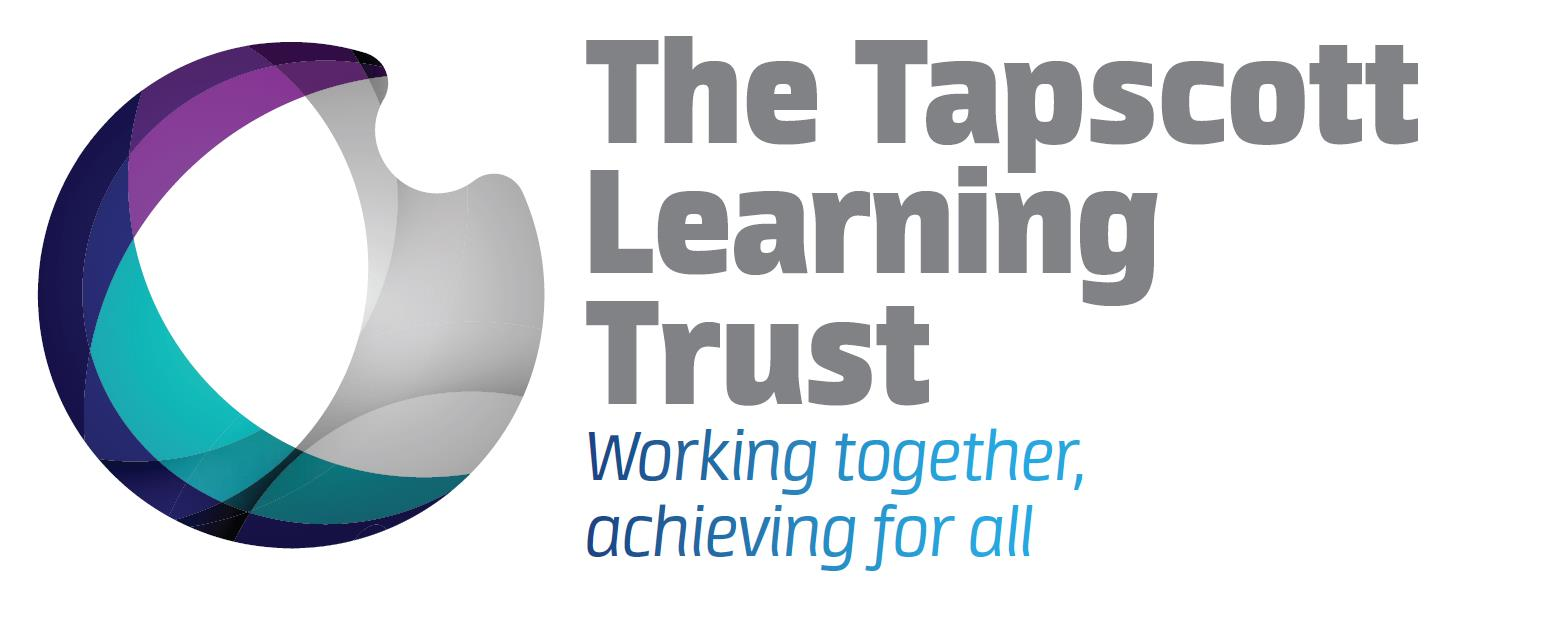••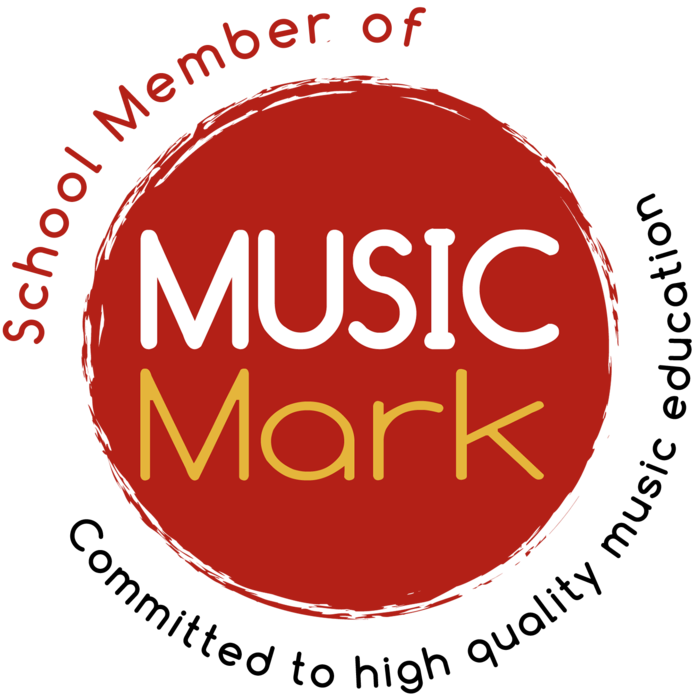••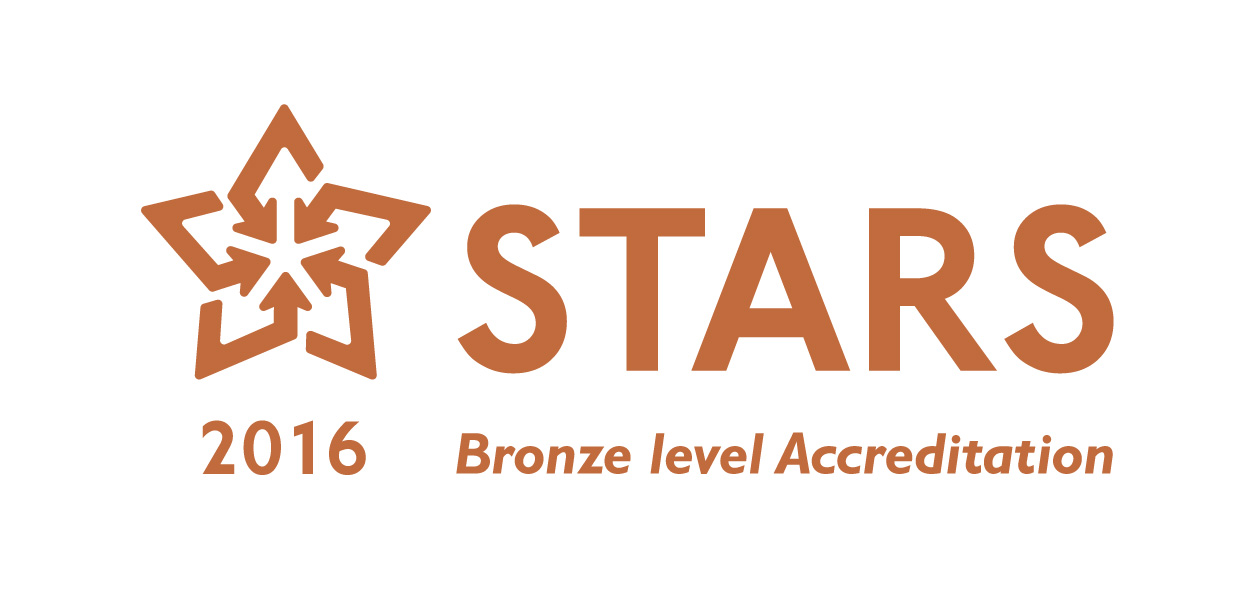•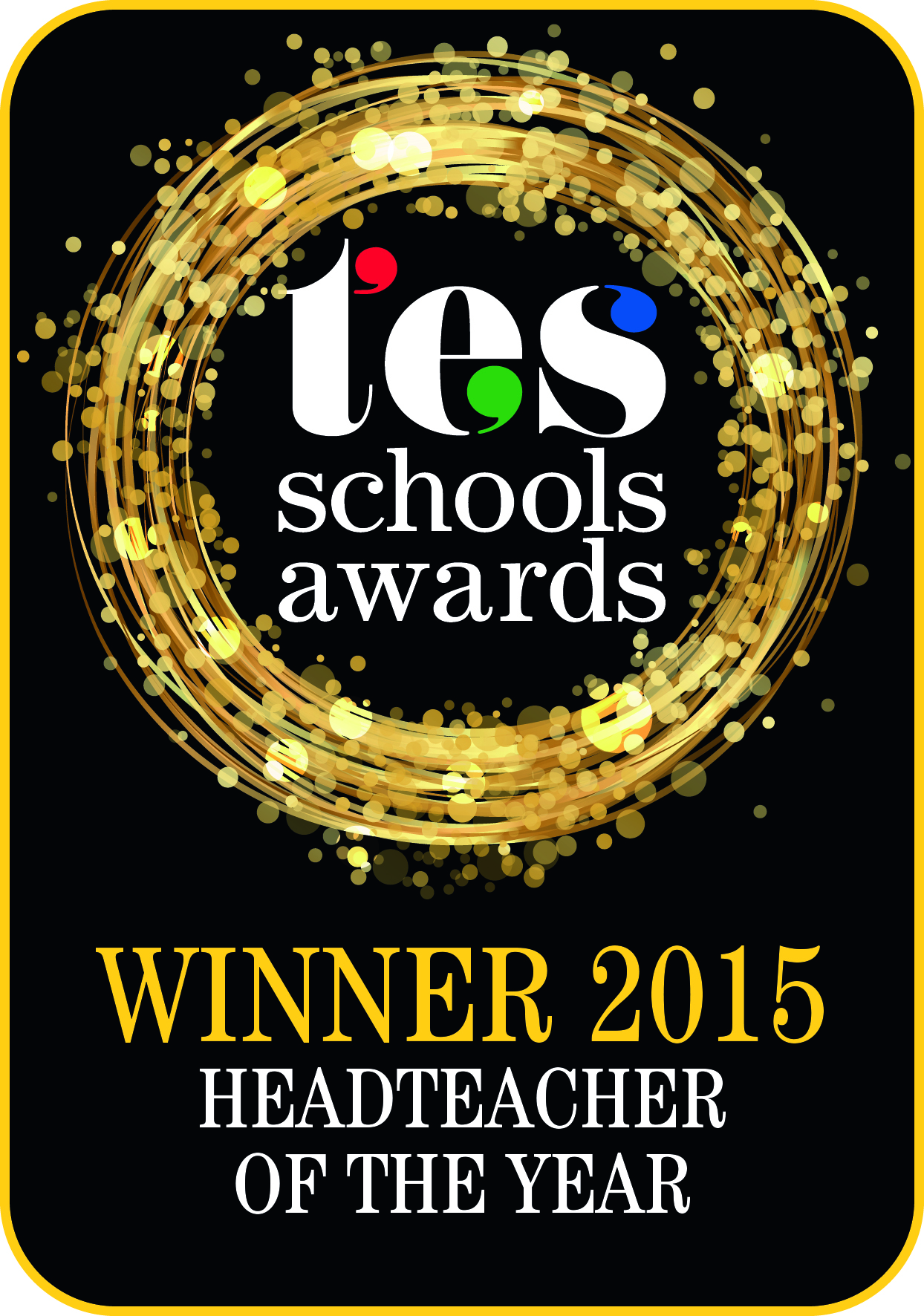•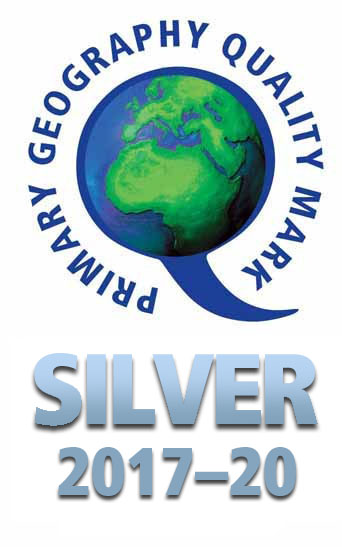•••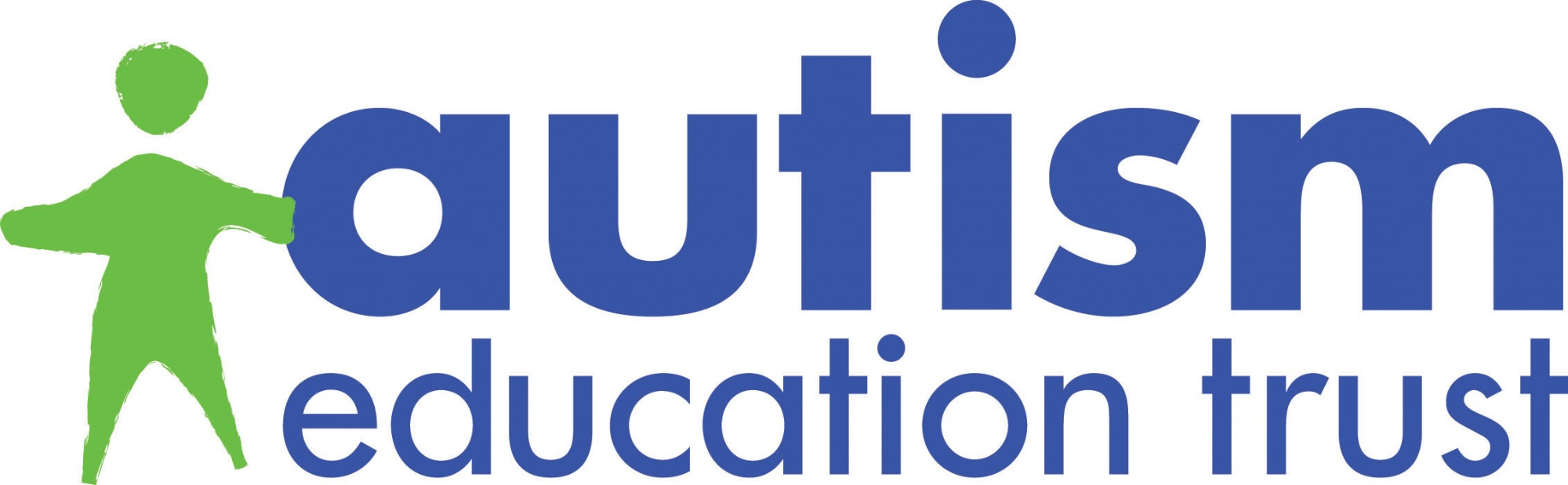•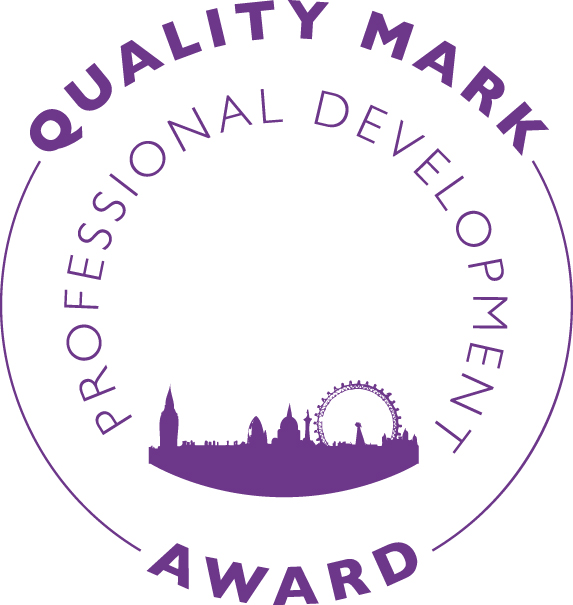••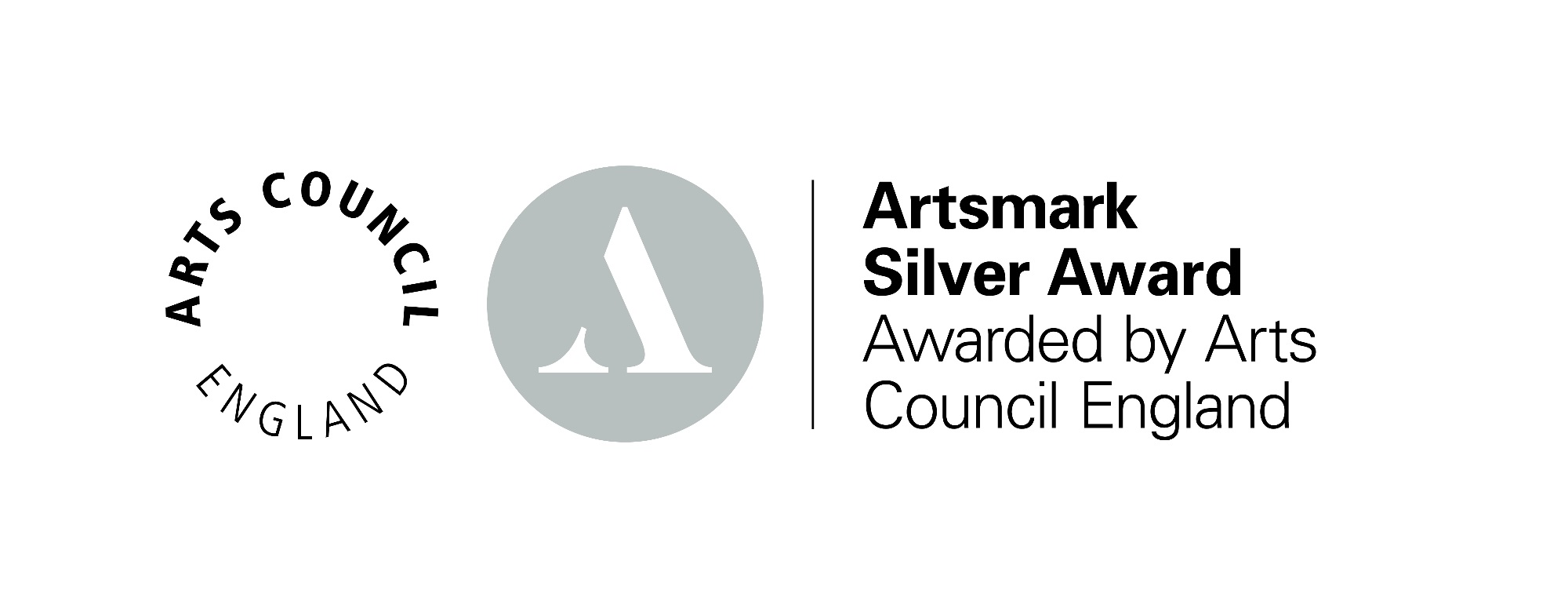•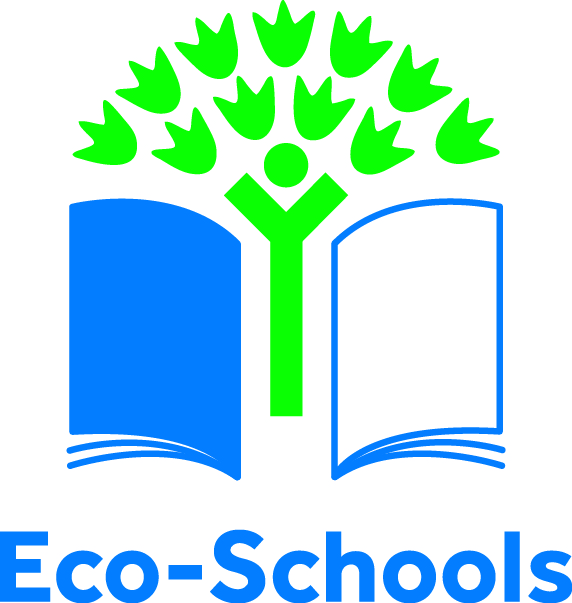••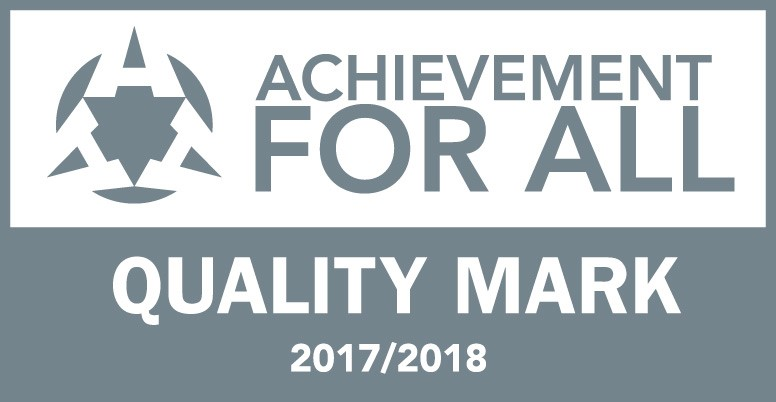•